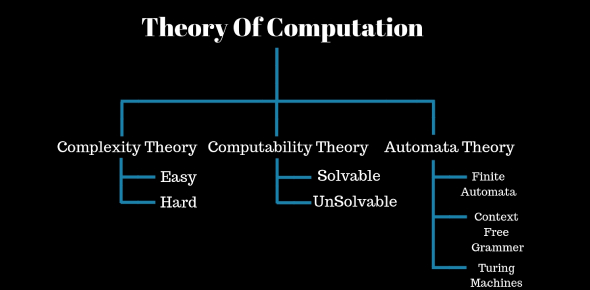# Theory Of Computation: Computer Science Trivia Quiz

10 Questions | Attempts: 914Settings.

• 1.
Computer Science is a cluster of related scientific and engineering disciplines concerned with the study and application of:
• A.

Computers

• B.

Compartments

• C.

Computations

• D.

Combinations

• 2.
The Theory of Computation is a scientific discipline concerned with the study of
• A.

General properties of combination be it relative to natural, complexity, or imaginary

• B.

General properties of computation be it natural, man-made, or imaginary

• C.

General properties of computation related to the study of difficulty aspects of variations

• D.

General properties of computation be it natural, handmade, or imagination

• 3.
The foundations (or theory) of computer science can be partitioned into two sub-disciplines: one concerned with the
• A.

Theory of Compilation with the Theory of Programming

• B.

Theory of Combination with the Theory of Probability

• C.

Theory of Computation with the Theory of Programming

• D.

Theory of Complexity with the Theory of Progressive

• 4.
The Theory of Computation is also concerned with finding the
• A.

Most worst case solutions for solving specific problems

• B.

Most efficient methods for solving specific problems

• C.

Most sufficient methods for solving non-specific problems

• D.

Most efficiently artificial methods for solving complex problems

• 5.
The nature of efficient computation (and computation in general) is indeed the formative question of the
• A.

Theory of Computation

• B.

Theory of Automata

• C.

Theory of Combination

• D.

Theory of Probability

• 6.
Form the most important class of automata between finite-state machines and Turing Machines.
• A.

Non-Deterministic Finite Automata

• B.

Deterministic Finite Automata

• C.

Pushdown Automata

• D.

Pushdown Accepter

• 7.
Comprises a finite-state control, a semi-infinite input tape, and a semi-infinite storage tape.
• A.

Deterministic Finite Automata

• B.

Pushdown Accepter

• C.

Pushdown Stack

• D.

Pushdown Automata

• 8.
The character empty null string(triangular shaped) is used to indicate a _____________ in a tape cell.
• A.

Bound

• B.

Lowercase letters

• C.

First blank cell

• D.

Blank

• 9.
is a place where input letters or other information can be stored until we want to refer to them again.
• A.

Pushdown Stack

• B.

Pushdown Store

• C.

A or B

• D.

None of the above

• 10.
Adds a new letter to the line and it is placed on top of the stack, and all other letters are pushed back or down accordingly.
• A.

Blank

• B.

Tape

• C.

Stack

• D.

Push

## Related TopicsBack to top
×

Wait!
Here's an interesting quiz for you.